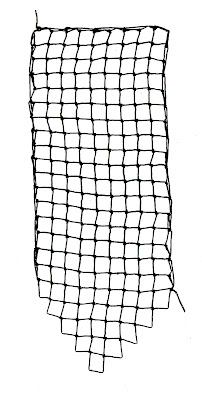## Thursday, July 2, 2020

### One Pointed End on a Rectangle of Square-mesh NettingLast summer I was working on square-mesh triangles. As I was checking the triangle patterns, I realized that I had never combined a rectangle with a triangle.

I discovered that if the width of the rectangle is odd, the triangle will have a single point. (If the width is of the rectangle is even, there will be two squares at the point, but that's a post for another day.)

By the time I finished the netting, I ran out of time to post them, so here they are, a year late.

Instructions for:

Rectangle of Square-mesh Netting – From One Corner with One Pointed End

Odd width 9 squares, Even length 18 squares, 5 points along pointed sides

Form the Rectangle Width
Row 1:  Net 2 knots in the foundation loop. (2 loops in the row)
Remove the mesh stick and turn the work so that the next row can be worked from left to right. (This will be done at the end of each row.)
Row 2:  Net 1 knot in the first loop on the left; increase by netting 2 knots in the second loop. (3 loops in the row)
Row 3:  Net 1 knot in each loop except the last loop; net 2 knots in that last loop. (4 loops in the row)
Row 4:  Net 1 knot in each loop except the last loop; net 2 knots in that last loop. (5 loops in the row)
Row 5:  Net 1 knot in each loop except the last loop; net 2 knots in that last loop. (6 loops in the row)
Row 6:  Net 1 knot in each loop except the last loop; net 2 knots in that last loop. (7 loops in the row)
Row 7:  Net 1 knot in each loop except the last loop; net 2 knots in that last loop. (8 loops in the row)
Row 8:  Net 1 knot in each loop except the last loop; net 2 knots in that last loop. (9 loops in the row)
Row 9: Net 1 knot in each loop except the last loop; net 2 knots in that last loop. (10 loops in the row)
Row 10:  Net 1 knot in each loop except the last loop; net 2 knots in that last loop. (11 loops in the row)
Lengthen the Rectangle
Row 11: (Decrease Row Net 1 knot in each loop until you come to the last 2 loops; net the last 2 loops together. (10 loops in the row)

Row 12: (Increase row): Net 1 knot in each loop except the last loop; net 2 knots in the last loop. (11 loops in the row)
Row 13: (Decrease Row Net 1 knot in each loop until you come to the last 2 loops; net the last 2 loops together. (10 loops in the row)

Row 14: (Increase row): Net 1 knot in each loop except the last loop; net 2 knots in the last loop. (11 loops in the row)
Row 15: (Decrease Row Net 1 knot in each loop until you come to the last 2 loops; net the last 2 loops together. (10 loops in the row)

Row 16: (Increase row): Net 1 knot in each loop except the last loop; net 2 knots in the last loop. (11 loops in the row)
Row 17 (Decrease Row): Net 1 knot in each loop until you come to the last 2 loops; net the last 2 loops together. (10 loops in the row)

Row 18: (Increase row): Net 1 knot in each loop except the last loop; net 2 knots in the last loop. (11 loops in the row)
Row 19: (Decrease Row): Net 1 knot in each loop until you come to the last 2 loops; net the last 2 loops together. (10 loops in the row)

Finish the Rectangle with a Lacy Point
Row 20: (Plain row): Net 1 knot in each loop. (9 loops in the row)

Row 21: (Decrease Row): Net 1 knot in each loop until you come to the last 2 loops; net the last 2 loops together. (8 loops in the row)

Row 22: (Plain row): Net 1 knot in each loop. 8 loops in the row)

Row 23: (Decrease Row): Net 1 knot in each loop until you come to the last 2 loops; net the last 2 loops together. (7 loops in the row)

Row 24: (Plain row): Net 1 knot in each loop. (7 loops in the row)

Row 25: (Decrease Row): Net 1 knot in each loop until you come to the last 2 loops; net the last 2 loops together. (6 loops in the row)

Row 26: (Plain row): Net 1 knot in each loop. (6 loops in the row)

Row 27: (Decrease Row): Net 1 knot in each loop until you come to the last 2 loops; net the last 2 loops together. (5 loops in the row)